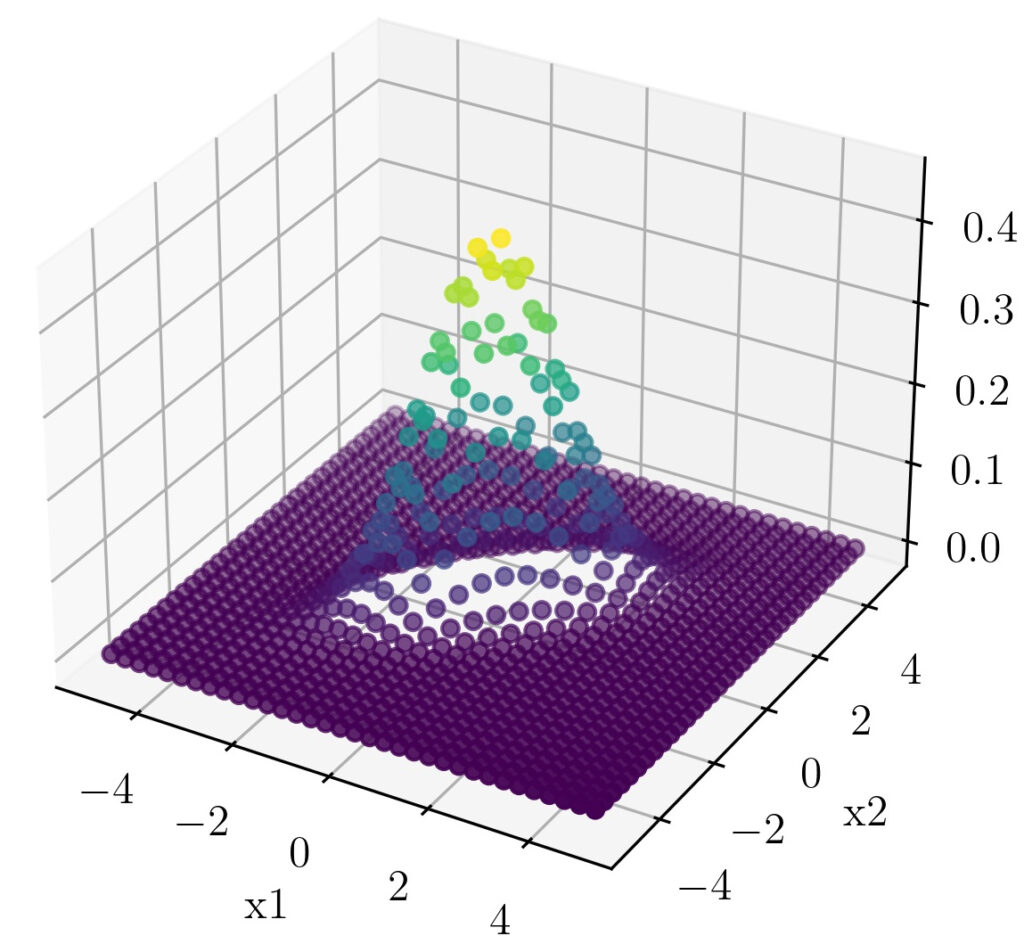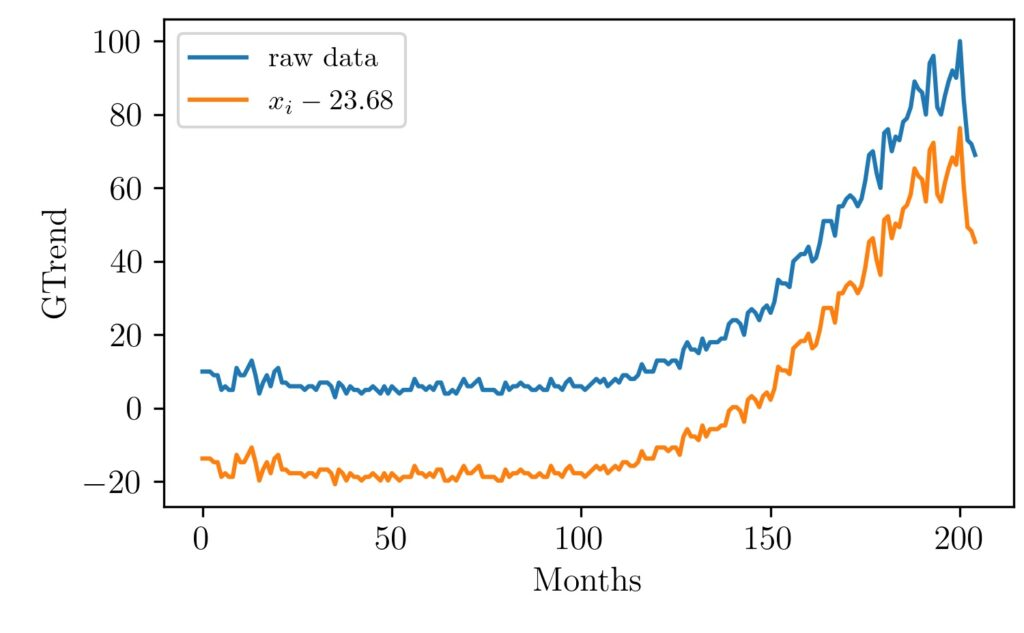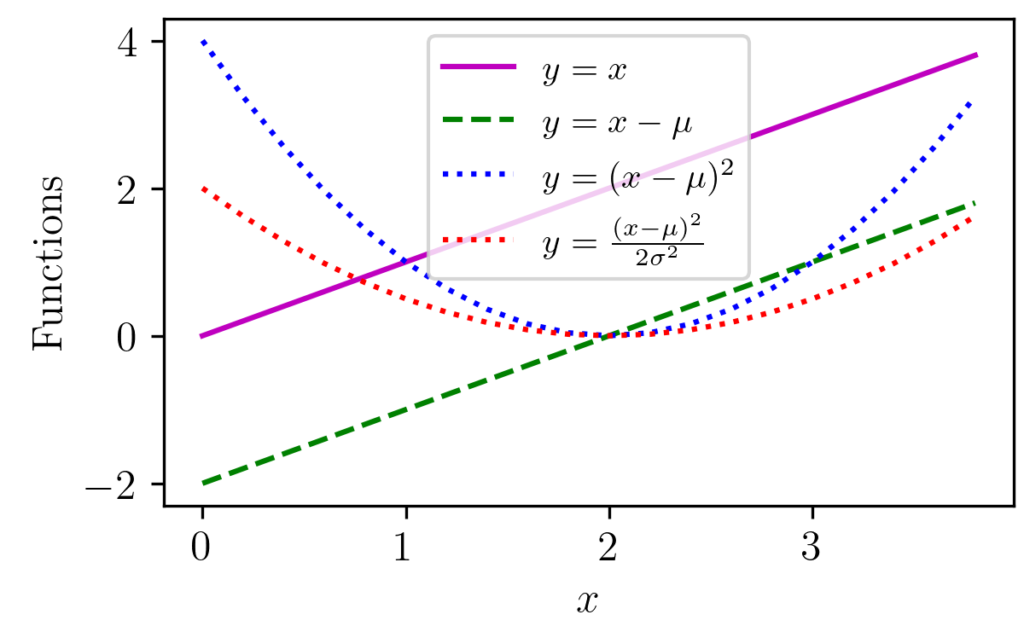# Month: January 2021

## The Art of Dividing and Conquering

Introduction Divide and conquer seem the most widely used strategy to attack hard problems. The use of this idea and similar approaches are ample in every discipline. In this post, let’s look at how this concept is a cornerstone in analyzing signals and systems. Consider the case of audio signals. We can think of them …## A World of small things: Introduction to Nanotechnology

Nanotechnology deals with the science of building small, but why do we talk about such small things? Well, they ultimately constitute the world we live in and we can explore fascinating things with them, literally, it can shape the world around us. Properties of materials change due to quantum effects when they are made small, …## Hacking the Bivariate Gaussian Distribution

In one of our earlier posts, we have seen how we can visually relate the parts of the one-dimensional Gaussian distribution equation. In this post, we will follow the same strategy to understand the terms that comes up with a Multivariable Gaussian distribution. We will focus on the Bivariate Gaussian distribution as distributions of higher-order …## Visualizing MinMax Scaling

Normalization of vectors, an array of values, signals is often used as a preprocessing step before many algorithms. For example, in machine learning, some types of algorithms are prone to different inherent scales of features. In such situations normalization is done to give the same weightage to all features before passing it to the algorithm …## Gaussian Distribution Explained Visually

Gaussian distribution appears in various parts of science and engineering. Apart from a distribution often appear in nature, it has got important properties such as its relation to Central Limit Theorem (CLT). The figure above shows one-dimensional Gaussian distributions of various mean and variance values. Libraries like NumPy provide functions that can return Gaussian distribution …